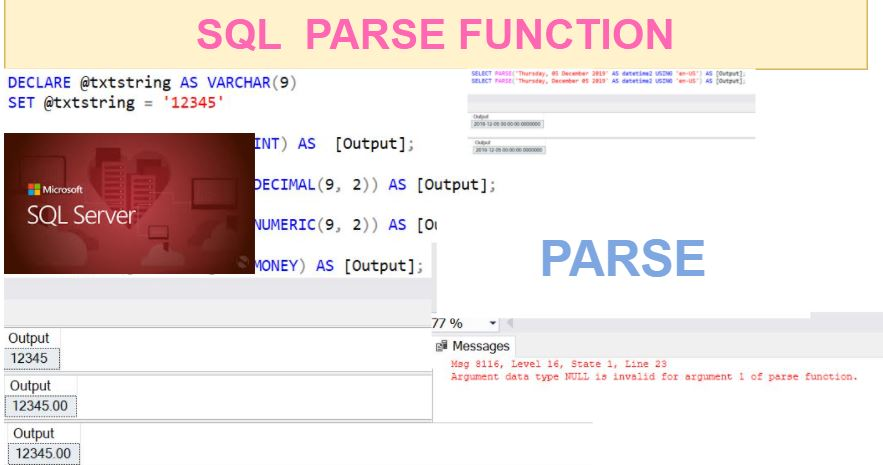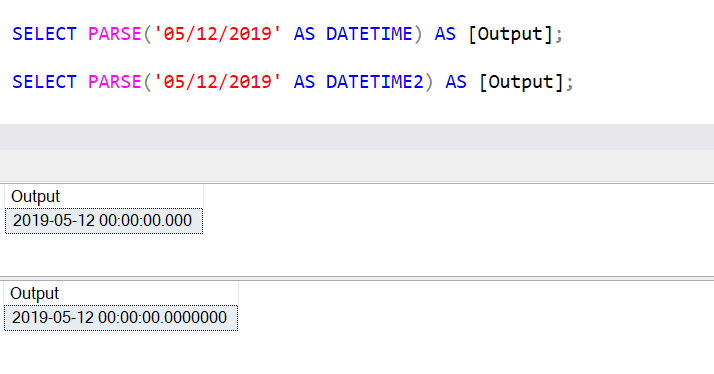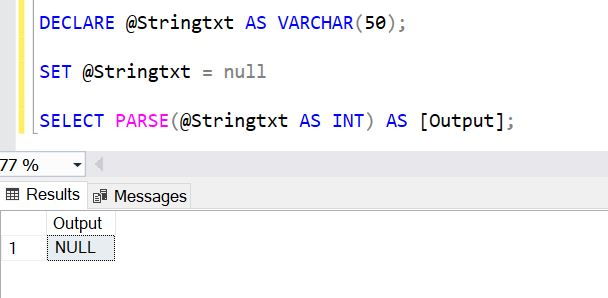Home » SQL PARSE Function

# SQL PARSE Function### The SQL PARSE function is a SQL Conversions Function that is used to convert the string data to the requested data type and returns the result as an expression. It was introduced to T-SQL in SQL Server 2012.

Parse function retuns an error, if it fails to convert the string into the requested data type, or any non convertible string or NULL value is passed to PARSE function.

Consider Parse Function only to convert the strings into date and time, and numeric values.

SYNTAX

`PARSE ( string_value AS data_type [ USING culture ] )`
• String_value: Is the value of the expression we want to change to a specific data type.
• Data_Type: Data Type to which you want to convert the string_value.
• Culture: Is an optional parameter. By default, it uses the current session language.

Lets look at an example using SQL PARSE function in SQL Server.

#### PARSE STRING TO NUMERIC

The following example using PARSE function to convert a specific string value to an Integer, Decimal, Numeric, and Money.

```DECLARE @txtstring AS VARCHAR(9)
SET @txtstring = '12345'

SELECT PARSE(@txtstring AS INT) AS [Output];

SELECT PARSE(@txtstring AS DECIMAL(9, 2)) AS [Output];

SELECT PARSE(@txtstring AS NUMERIC(9, 2)) AS [Output];

SELECT PARSE(@txtstring AS MONEY) AS [Output];

```#### PARSE STRING TO DATETIME and DATETIME2

The following example using PARSE function to convert a specific string value to DATETIME, and DATETIME2.

```SELECT PARSE('05/12/2019' AS DATETIME) AS [Output];

SELECT PARSE('05/12/2019' AS DATETIME2) AS [Output];```#### PARSE a string value into a datetime2, using a specific language value

```SELECT PARSE('Thursday, 05 December 2019' AS datetime2 USING 'en-US')AS [Output];
SELECT PARSE('Thursday, December 05 2019' AS datetime2 USING 'en-US')AS [Output];```

The USING statement that is used in above statement allows to specify the culture, or language the string value is formatted in.#### PARSE WITH NULL VALUES

If a constant NULL values is passed to PARSE function it gives an error as shown below.

`SELECT PARSE(null AS INT) AS [Output];`If a parameter with a null value is passed, then it returns null as shown below.

```DECLARE @Stringtxt AS VARCHAR(50);

SET @Stringtxt = null

SELECT PARSE(@Stringtxt AS INT) AS [Output];```